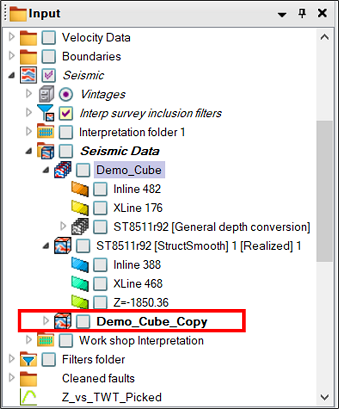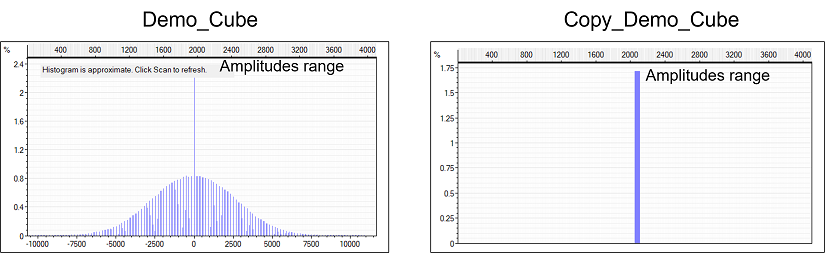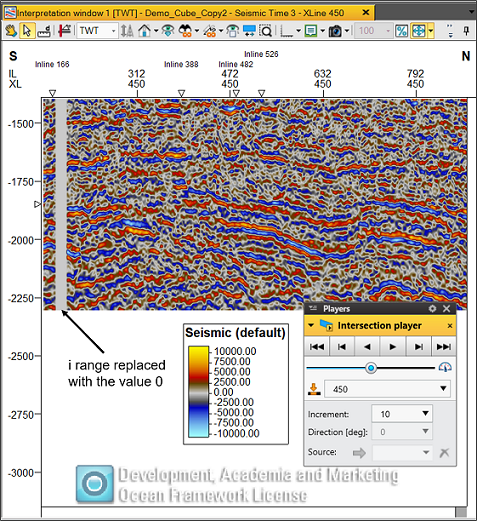# Seismic#

This section offers an overview of the different functions and properties available for the Petrel Seismic object subcategories.

## Seismic Cube#

Check out the API documentation to view a detailed description of all the functions and properties available for Seismic Cubes.

To retrieve all the seismic cubes from our Petrel project we can use the .seismic_cubes property. This property returns all the seismic cubes within the project in a dictionary where the keys represent the path of the cube within the Petrel input tree and the value represents the name of the cube:

:

from cegalprizm.pythontool import PetrelConnection
petrel = PetrelConnection()

all_cubes = petrel.seismic_cubes
print(all_cubes)

{'Input/Seismic/Seismic Data/Demo_Cube/ST8511r92 [General depth conversion]': Seismic(petrel_name="ST8511r92 [General depth conversion]"), 'Input/Seismic/Seismic Data/Demo_Cube': Seismic(petrel_name="Demo_Cube"), 'Input/Seismic/Seismic Data/ST8511r92 [StructSmooth] 1 [Realized] 1': Seismic(petrel_name="ST8511r92 [StructSmooth] 1 [Realized] 1")}


Using a for loop, we can iterate through the dictionary and print out all the seismic cubes names:

:

for cube in petrel.seismic_cubes:
print(cube.petrel_name)

ST8511r92 [General depth conversion]
Demo_Cube
ST8511r92 [StructSmooth] 1 [Realized] 1


Let’s select the Demo_Cube seismic cube. First, we save all the paths of the cubes to a list, the we can slice through the list and assign the selected value to the Demo_Cube variable:

:

cube_path = list(all_cubes.keys())
Demo_Cube_path = cube_path
Demo_Cube=petrel.seismic_cubes[Demo_Cube_path]
print(Demo_Cube.petrel_name)

Demo_Cube


We can obtain the size of the grid by using the .extent property, which will return the number of nodes in the i, j and k directions. Seismic traces are indexed by i and j, with k specifying the sample number in a trace:

:

extent = Demo_Cube.extent
print(extent)

Extent(i=375, j=301, k=227)


The .coords_extent returns the extent of the seismic cube in world-coordinates (Xmin, Xmax, Ymin, Ymax, Zmin, Zmax):

:

coord_extent = Demo_Cube.coords_extent
print(f'x: {coord_extent.x_axis}')
print(f'y: {coord_extent.y_axis}')
print(f'z: {coord_extent.z_axis}')

x: AxisExtent(min=451187.180000, max=458842.610000)
y: AxisExtent(min=6780245.450000, max=6789707.300000)
z: AxisExtent(min=-2304.720000, max=-1396.000000)


The .has_same_parent() function checks if two cubes belong to the same parent collection and returns a boolean value. In the example below, we assign the other two cubes in our dictionary to the variables Cube0 and Cube1:

:

Cube0 = petrel.seismic_cubes[cube_path]
Cube1 = petrel.seismic_cubes[cube_path]
print(f'{Cube1} and {Demo_Cube} has same parent: {Cube1.has_same_parent(Demo_Cube)}')
print(f'{Cube0} and {Demo_Cube} has same parent: {Cube0.has_same_parent(Demo_Cube)}')

Seismic(petrel_name="ST8511r92 [StructSmooth] 1 [Realized] 1") and Seismic(petrel_name="Demo_Cube") has same parent: True
Seismic(petrel_name="ST8511r92 [General depth conversion]") and Seismic(petrel_name="Demo_Cube") has same parent: True


Using the .indices() and .position() functions we can obtain the centre of a seismic node with its coresponding indices. First, we assign the indices of a seismic node to the node_indices variable. For the indices parameters, we are using the i,j,k extents of the node and then use floor division to get the i,j,k of centre of the node. Once we have the i,j,k of the centre, we can then use the .position() function to get the XYZ position of the node centre based on the provided i,j,k parameters:

:

from cegalprizm.pythontool.primitives import Indices
from cegalprizm.pythontool.primitives import Point

node_indices = Indices(i = extent.i//2, j = extent.j//2, k = extent.k//3)
node_center_position = Demo_Cube.position(node_indices.i, node_indices.j, node_indices.k)
print(f'{node_indices}: {node_center_position}')

Indices(i=187, j=150, k=75): Point(x=455014.90, y=6784976.38, z=-1698.24)


Alternatively, we can obtain the indices of a seismic node at a specified XYZ position:

:

pos = node_center_position
node_indices_at_pos = Demo_Cube.indices(pos.x, pos.y, pos.z)
print(f'{pos}: {node_indices_at_pos}')

Point(x=455014.90, y=6784976.38, z=-1698.24): Indices(i=187, j=150, k=75)


The .annotation() function returns the annotations for seismic indices with inline, xline and sample of the given index(i,j,k directions, k-defaults to 0):

:

Demo_Cube.annotation(30,60)

:

Annotation(inline=212, xline=270, sample=1)


Similarly, the .annotation_indices() function returns the i,j and k index of a particular inline, crossline and sample number(defaults to 1):

:

Demo_Cube.annotation_indices(212,260)

:

Indices(i=30, j=55, k=0)


We can create a chunk that contains all the values in the entire seismic cube by using the .all() function. This can be an expensive operation in time and memory depending on the size of cube. To learn more about chunks please view the Working with chunks subchapter of the User Guide.

[ ]:

Demo_Cube.all()


Alternatively, we can create a chunk containing values in the ranges specified by using the .chunk() function:

:

cube_chunk = cube.chunk((0,1), (1,3), (0,3))
print(cube_chunk)

Chunk(backing_object=Seismic(petrel_name="ST8511r92 [StructSmooth] 1 [Realized] 1"), i=(0, 1), j=(1, 3), k=(0, 3))


Using the .clone() function, we can create a copy of the seismic cube. The copy_values parameter is optional and requires a boolean value. If the copy_values parameter is set to True, the values of the cube will be copied into the clone. By default, the copy_values is set to False, and the values are not copied to the clone. The cloned cube is placed in the same collection as the source object. A clone cannot be created with the same name as an existing Petrel object in the same collection::

Demo_Cube.clone('Demo_Cube_Copy',copy_values=True)

:

Seismic(petrel_name="Demo_Cube_Copy")


The set_value() function sets the values of the entire seismic cube to the value provided. This is useful to set all values to zero, but any value can be provided. The following example sets the value of the cloned cube (Demo_Cube_Copy) to 0::

# Select the Demo_Cube_Copy:

all_cubes = petrel.seismic_cubes
cube_path = list(all_cubes.keys())
Copy_Demo_Cube_path = cube_path
Copy_Demo_Cube=petrel.seismic_cubes[Copy_Demo_Cube_path]

# Set the readonly value of the cube to False so we can modify its values

#set all the values to 0
Copy_Demo_Cube.set_value(0)

:

True


Using .columns() function we can set all the amplitude values to a specific values across a range of i and j-slices. In the following example we replaced all the amplitudes values within the i-slices i=10 through i=19 with the 0 value. Before it we’ve set the readonly value of the seismic cube to false so we can modify the values. If we don’t specify the range it will replace all values. The results are written to Petrel in real time:[ ]:

Demo_Cube.readonly=False
for col in Copy_Demo_Cube.columns(range(10, 20), jrange=None):
col.set(0)


The .reconnect() function reconnects a 3D seismic object to the given file path.The file path has to be written with 2 backslashes. This method only works on external seismic objects with broken links: